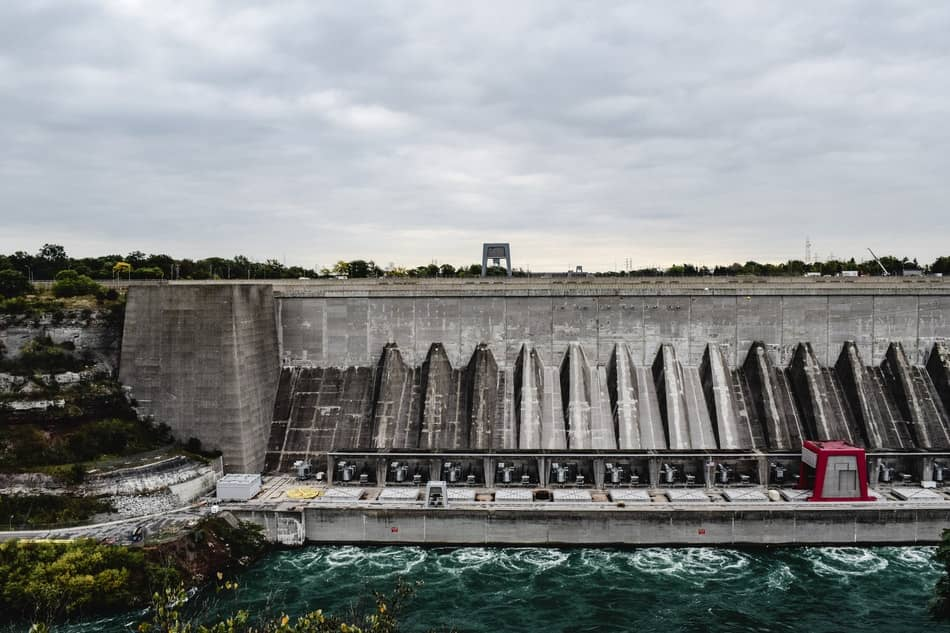Back to Hydro Energy

# What is Hydropower?One of the first questions which come to mind when people hear about Hydropower is: What is Hydropower? It takes some reading and some analysis of the word to understand and the importance of Hydropower in our lives. Hydropower is one of the Renewable Energy Sources, RES, and as a matter of fact is the largest RES in terms of electricity production. In some cases Hydropower is called hydroelectric power as the most common use of Hydropower is the production of electricity.

In order to understand Hydropower and its use we will provide the definition and a high level analysis of certain terms which are used in the Hydropower field.

### Hydropower

Hydropower is basically the power of water. It can be the power of running water or the power of falling water. Running or falling water contains energy and the power derived from this energy can be captured to produce useful work. During our times the useful work that we mean is the production of electricity and thus the term hydroelectric power. Historically hydropower was used for other useful work as well.

Running or falling water has energy and this is called Kinetic Energy. Kinetic Energy is the energy of motion, whether this motion is vertical or horizontal and it is the kind of energy which can produce useful work such as electricity.

Stored water or contained water has potential energy. For instance water stored in a dam or another storage device like a tank, contains potential energy which potentially can used to produce useful work needed such as electricity. However in order for this energy to be useful it needs to be converted to kinetic energy and one way to do this is by letting the water flow and fall from a height thus transforming it to kinetic energy.

### How much power does water have?

The power of water is called Hydropower and it is a function of two parameters: the rate of flow of water and the water’s head or hydraulic head.

The rate of flow of liquid is the volume of liquid which passes through a given point or through the system per unit of time. Thus the rate of flow of water in seconds is the volume of water it passes through the system is one second and it can be given in terms of cubic feet per second or in the metric system in cubic meters per second. The rate of flow is applicable for both falling and running water.

The hydraulic head is the energy per unit mass of water. This head has a different definition for falling water and running water. The head for falling water is called static head and is proportional to the height difference of the falling water, in other words the height through the water falls. Dynamic head we call the head of running water which is a function of the velocity of the moving water.

The amount of work done per unit of water is the product of its weight and the head.

### The Hydro cycle

As it was mentioned many times Hydropower energy is a renewable energy source. This is due to the fact that water flow is renewed by following the hydro cycle. The hydro cycle is simple and follows a 6 step flow.

• Solar Energy heats the water in the oceans
• Water evaporates in the air
• The vapour condenses and becomes rain
• Rains falls back to the land
• The water flows into rivers
• Rivers flows into the oceans

The cycle repeats itself and thus hydropower energy is renewable since it is  produced from a constantly renewed source.

### How Hydropower works

There are many ways to capture hydropower but whichever way or method is used there are 4 main steps and 2 main components involved in the generation of electricity. The 2 main components are the turbine and the generator and the 4 steps are:

• Water flows through a water turbine. It is actually directed into the water turbine
• The energy or the force of the water spins the turbine
• The turbine is connected to a generator
• The generator produces electricity

### Hydroelectric Dam

As the name implies a hydroelectric Dam is a dam which is appropriately exploited, designed and operated, so as to produce electricity which both clean and renewable. There are about 80.000 dams in the USA but only 2.5% or 2000 of them are used for the production of electricity.

The dam collects and stores water and acts as a water reservoir. Stored water is released through specially designed spillway gates, flows through certain pipes or channels in a certain speed and spins the turbine which is connected to the generator for the production of electricity.

Hydroelectric power is by far the number one renewable energy source which produces 20% of the world’s electricity. In the US the production of electricity from hydropower is only 6% while in Norway it accounts for the amazing 99%. Some of the Hydropower facts and figures are listed in our article Hydropower and Hydroelectric Energy Facts and Figuresand the importance of Hydropower is presented.